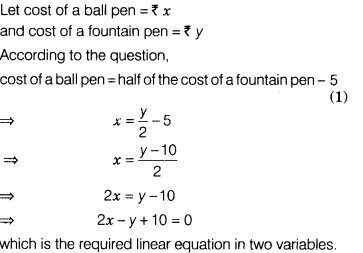# The cost of a ball pen is Rs.5 less than half of the cost of a fountain pen. Write this statement as a linear equation in two variables

The cost of a ball pen is Rs .5 less than half of the cost of a fountain pen. Write this statement as a linear equation in two variables.